Stoichiometry

Content Author: Mr. Friedrichs

Please note that the videos throughout this module will work best if viewed in Chrome or Firefox browsers.  Enjoy!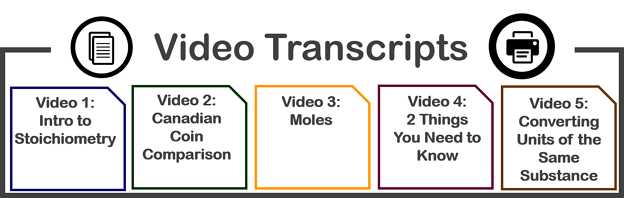# Problem Type 1

Convert mol of A (known) into mol of B (unknown)

 Introduction to Problem Type 1 (0:34)Type 1 (1:29)Type 1 problems require only 1 step to solve.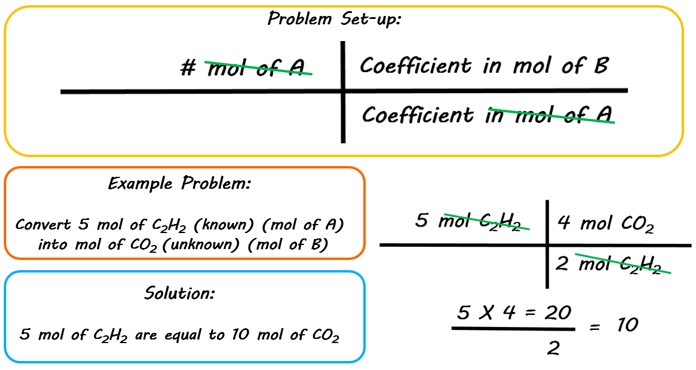# Problem Type 2

Convert mol of A (known) to grams of B (unknown)

 Introduction to Problem Type 2 (0:34)Type 2 (1:45)This type of problem requires only 2 steps to solve.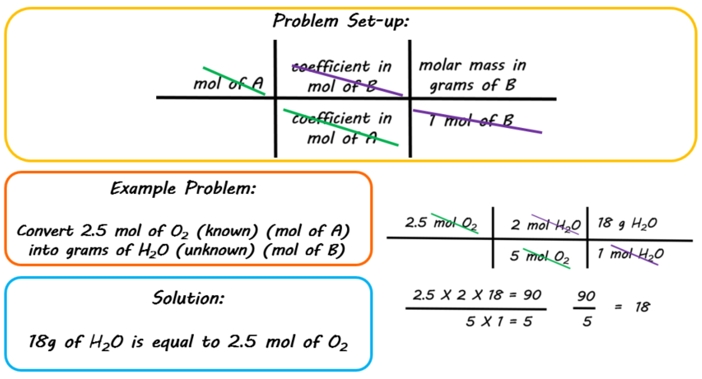# Problem Type 3

Convert grams of A to mols of B

 Introduction to Problem Type 3 (0:26)Type 3 (1:58)This type of problem requires only 2 steps to solve. It is just like problem type 2, except the steps are performed in the opposite order.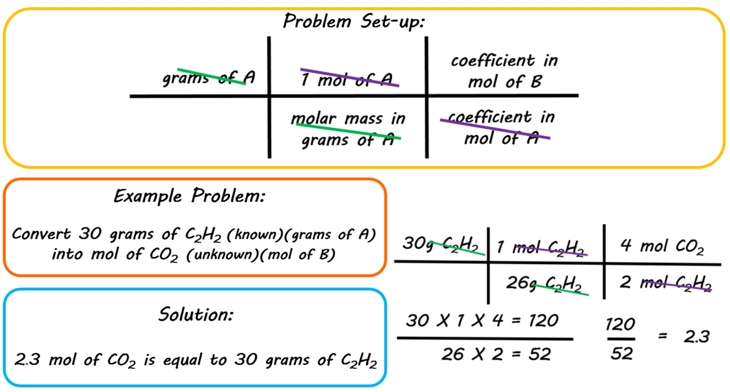# Problem Type 4

Convert grams of A to grams of B

This is the most common type of stoichiometry problem that you will encounter.

 Introduction to Problem Type 4 (0:28)Type 4 (2:16)This type of problem takes 3 steps to solve. You will combine together some of the earlier steps, but they will be performed in a different order.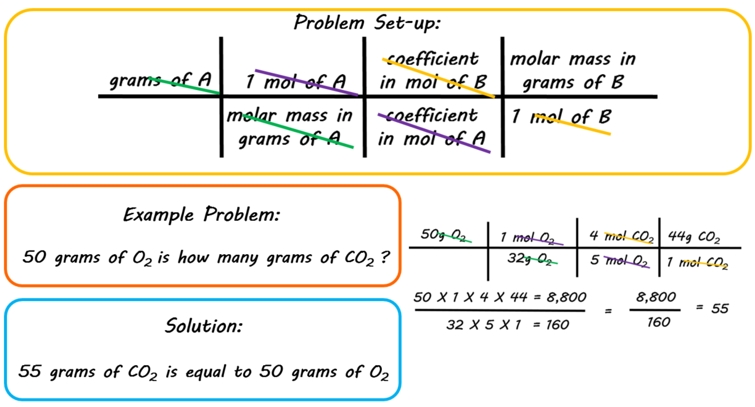# Give it a try!

Instructions: Click on the correct mole ratio from the choices on the right to complete the t-chart.